# Pool

The swimming pool is 10 m wide and 8 m long and 153 cm deep. How many hectoliters of water is in it, if the water is 30 cm below its upper edge?

Correct result:

V =  984 hl

#### Solution: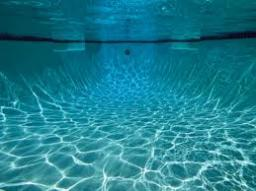We would be very happy if you find an error in the example, spelling mistakes, or inaccuracies, and please send it to us. We thank you!Math student
IT'S 9 CM NOT 10 CM. SO IT SHOULD BE 4004.Dr Math
corrected, thank youTips to related online calculators
Do you want to convert length units?
Do you know the volume and unit volume, and want to convert volume units?

## Next similar math problems:

• CalculateCalculate the surface and volume of the cone that results from the rotation of the right triangle ABC with the squares 6 cm and 9 cm long around the shorter squeegee.
• AquariumHow many liters of water can fit in an aquarium measuring 30, 15 and 20 cm?
• Wooden bowls20 wooden bowls in the shape of a truncated cone should be painted on the outside and inside with wood varnish. We need 0.1 l of paint to paint 200 cm2. How many liters of paint do we have to buy if the bowls are 25 cm high, the bottom of the bowl has a d
• Diver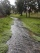Please calculate using Pascal's law. The window of the diving helmet has a surface content of about 7dm2. Calculate what pressure force acts on the window at a depth of 20 meters below the water surface.
• Two vases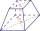Michaela has two vases in her collection. The first vase has the shape of a cone with a base diameter d = 20 cm; the second vase has the shape of a truncated cone with the diameter of the lower base d1 = 25 cm and with the diameter of the upper base d2 =
• Fresh juiceThe seller offers fresh-squeezed juice, which the customer either pours into his own containers or sells it in liter plastic bottles, which the customer buys from him. A liter of juice costs 40 CZK more than a bottle. There is no charge for turning the ju
• Triangular pyramidCalculate the volume of a regular triangular pyramid with edge length a = 12cm and pyramid height v = 20cm.
• Concrete columnThe concrete column of the highway bridge has the shape of a block with dimensions of 1m x 0.8m x 25m. It should be lifted by crane to a height of 20m. What is the power of his engine if the lifting takes 2 minutes?
• Pentagonal pyramidFind the volume and surface of a regular pentagonal pyramid with a base edge a = 12.8 cm and a height v = 32.1 cm.
• From plasticine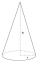Michael modeled from plasticine a 15 cm high pyramid with a rectangular base with the sides of the base a = 12 cm and b = 8 cm. From this pyramid, Janka modeled a rotating cone with a base diameter d = 10 cm. How tall was Janka's cone?
• Cuboid diagonalsThe cuboid has dimensions of 15, 20 and 40 cm. Calculate its volume and surface, the length of the body diagonal and the lengths of all three wall diagonals.
• Find theFind the surface area of a regular quadrilateral pyramid which has a volume of 24 dm3 and a height of 45 cm.
• Ice floe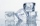What is the volume of an ice floe weighing 326 kg? The density of ice is only 920 kg/m3.
• The bodyThe body has dimensions of 2m 2dm and 10 cm. It weighs 28 kg. What is its density?The height of a regular quadrilateral prism is v = 10 cm, the deviation of the body diagonal from the base is 60°. Determine the length of the base edges, the surface, and the volume of the prism.Calculate the surface of the cone if its height is 8 cm and the volume is 301.44 cm3.Calculate the volume of the cone if the content of its base is 78.5 cm2 and the content of the shell is 219.8 cm2.